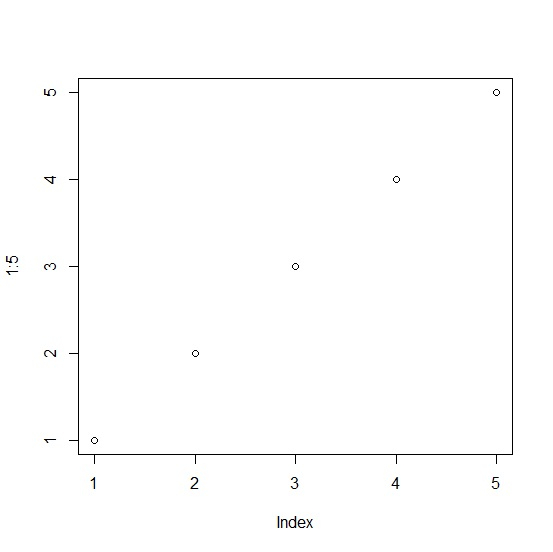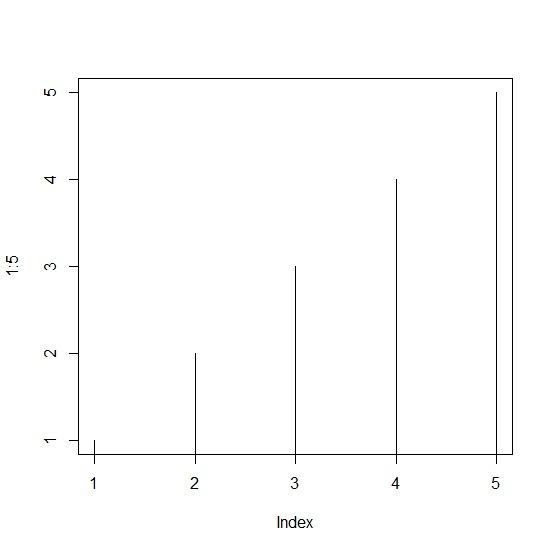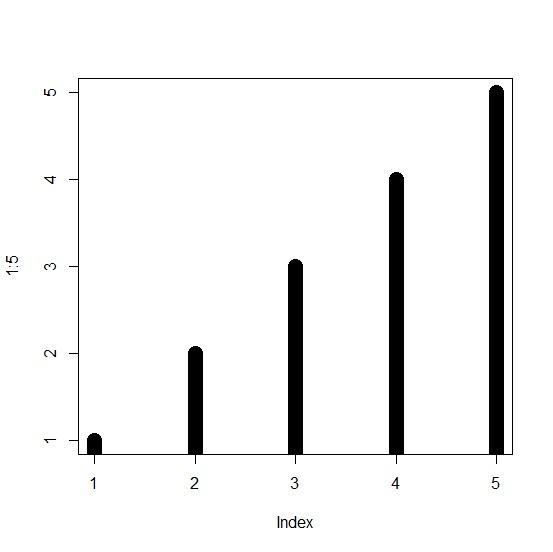# How to increase the width of lines for histogram like plots in base R?

The histogram like plot in base R is the plots of vertical lines instead of points in a vector or column of a data frame. If we increase the width of these lines then the plot becomes more like a histogram as the increment in widths make the vertical lines look like bars.

To increase the width of lines for histogram like plots in base R, we can use lwd argument.

Check out the below example to understand the difference between point chart and histogram like plot in base R.

## Example

Use the code given below to increase the width of lines for histogram like plots in base R −

plot(1:5)

## Output

If you execute the above given code, it generates the following output −For the histogram like plot, add the following code to the above snippet −

plot(1:5,type="h")


## Output

If you execute all the above given snippets as a single program, it generates the following output −For histogram like plot with larger width of lines, add the following code to the above snippet −

plot(1:5,type="h",lwd=15)

## Output

If you execute all the above given snippets as a single program, it generates the following output −Updated on: 05-Nov-2021

280 Views